# Understanding Motion

If you've ever studied physics, you'll know that motion is the result of forces acting on an object. In order for an object to move or to change direction, a force needs to be applied. We all know about the force of gravity, which pulls objects to the ground, but there are plenty of other forces that affect the way characters move, including wind, the weight of a heavy object, or even the forces exerted by a character's own muscles. A strong character moves much differently than a weakling does.

Motion is an object's change in position over time.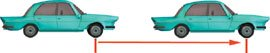When an object moves farther in the same amount of time, it has a higher velocity.Acceleration is a change in velocity.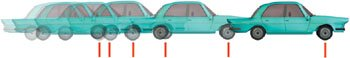Deceleration is another change in velocity, but in the opposite direction.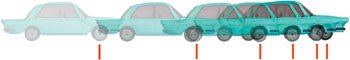Gravity and Other Forces

The most constant force in our world is that of gravity; if you live on Earth, or any other planet for that matter, you can't escape it. Knowing how gravity works will give you a good understanding of how all forces work.

Back in the 1600s, Isaac Newton figured out a mathematical formula to describe the effects of gravity and the laws of motion. I'm not going to bore you with equations in this book, but the basic idea is that applying a constant force to an object will cause it to accelerate. So an object subjected to the constant force of gravity will move faster and faster the longer it falls (of course, that's ignoring all other forces, such as wind resistance).

A constant force of gravity is applied to this bowling ball.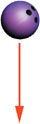Since animation is a visual medium, let's visually depict how a force affects an object.

The force of gravity causes the ball to accelerate. This means that it moves farther in each frame.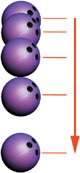When the bowling ball is tossed, the force of gravity accelerates the vertical motion in the same manner, though the horizontal motion is the same.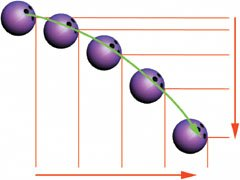For an object tossed upward, gravity causes its upward motion to decelerate until it comes to a halt, at which point the object accelerates downward. The resulting motion traces out a parabola.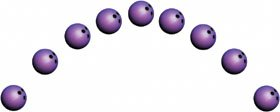These rules apply not just for gravity, but for every other force we encounter. An automobile applies force to the ground by turning its wheels. If this force is constant, the car will accelerate and push the driver back in his seat in much the same way that gravity pulls him down.

Muscle Forces

A character's muscles also apply force. Muscles can exert a force outward, such as when lifting an object, but they also work to move the body itself. A character's muscles constantly apply force to the skeleton to keep the character standing or to make the character perform actions. These muscle forces work just the same as gravity in that they cause objectsin this case, the character's limbsto accelerate and decelerate.

The fact that muscles apply force is a very important concept. Forces cause things to accelerate, and characters almost always accelerate into their motions and decelerate out of them. As an artist, you don't need to understand all the physics involved; you simply need to know that it takes things a while to get moving and that it also takes a while for things to stop. In animation lingo, this is known as a slow-in and slow-out.

The force applied by a character's bicep bends the arm at the elbow. If this force is constant, the end of the arm accelerates as it moves.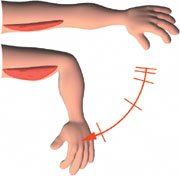The force applied by the triceps works in the opposite direction and decelerates the forearm, slowing it to a stop.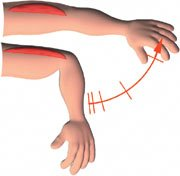Momentum and Weight

For objects to appear to be real, they need to have weight. An object in a 3D application has no inherent weight. The animator is the one who gives the illusion of weight by animating that object in a specific way. The motion of the object communicates a feeling of weight to the audience.

As we have seen, the forces acting upon an object determine how the object moves. Another factor that affects how the object moves is the mass of the object itself. This is called momentum, and what it means is that the more massive an object is, the more force is needed to change that object's direction. This rule is pretty much common sense: it's much easier to stop a bicycle than it is to stop a freight train. When animating objects, you need to pay attention to this little fact of life.

Here's a simple example. We have a ball. Is it filled with air or lead? The only way to tell is by how the ball moves.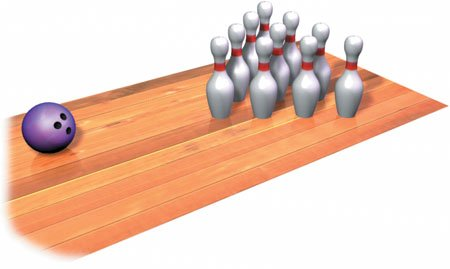In this example, the ball and the contents of the scene are identical. The only difference is the motion of the ball and pins. The ball's motion shows the audience the weight of the ball. Simply by changing the ball's motion, we turn it from a bowling ball into a balloon.

The ball is set in motion and rolls towards the pins.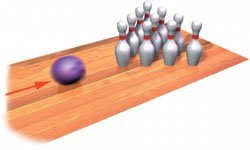If the ball crashes through the pins, we know that it is heavy and had lots of momentum.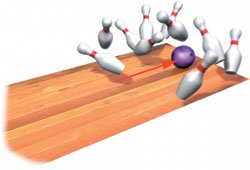If the ball bounces off the pins, we know that it is lighter and had less momentum than the first ball.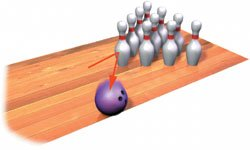If the ball then floats upward, we see that it is filled with helium.Chapter One. Basics of Character Design

Chapter Two. Modeling Characters

Chapter Three. Rigging Characters

Chapter Four. Basics of Animation

Chapter Five. Creating Strong Poses

Chapter Six. Walking and Locomotion

Chapter Seven. Facial and Dialogue Animation

Chapter Eight. Animal Motion

Chapter Nine. Acting

Chapter Ten. Directing and Filmmaking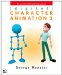Digital Character Animation 3 (No. 3)
ISBN: 0321376005
EAN: 2147483647
Year: 2004
Pages: 84